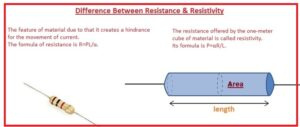Hello, friends, I hope you all are doing great. In today’s tutorial, we will discuss the Difference Between Resistance & Resistivity. The basic difference between resistance and resistivity is that resistance restricts the movement of current and resistivity is a feature of substance that discuss the resistance offered by the substance with certain dimensions.

In today’s post, we will have a detailed look at both resistance and resistivity and compare them to find their differences. So let’s get started with a Difference Between Resistance & Resistivity.

#### Difference Between Resistance & Resistivity

Resistance

• The feature of material due to that it creates a hindrance for the movement of current.
• The formula of resistance is R=ΡL/α.
• The resistance is a term used in the electrical system to explain the opposition that occurs in the flow of current
• Its measuring unit is ohm denoted with omega
• By using the relation among the three terms volts, current, and resistance ohm law was explained. That says V=IR
• The substance that offed the less value of resistance to flow current called conductor and that offed high value of resistance called the insulator
• High resistance less current flow low resistance high current will flow
• V=IR
• A large portion of any substance define the resistance offed by that material
• Rubber has less high resistance since it do not have free electrons
• The size and shape of the substance also define the resistance offered.
• If the area of the conductor is less and the length is high it has a large resistance.
• Such substance that has 0 value of resistance called superconductor
• The system international unit fo resistance is the ohm,
• It is denoted with the R.The main elements that affect the resistance are the length of the conductor, the cross-section area of the conductor, and temperature.Resistivity

• The resistance offered by the one-meter cube of material is called resistivity.
• Its formula is Ρ=αR/L.
• α is an area of the conductor.
• R is a resistance of substance.
• L is the length of the material.
• Resistivity is called volume resistivity is the resistance offered by the one-meter cube of substance.
• Its inverse is known as conductivity.
• Less value of resistivity indicates current can pass through a substance
• Resistivity is denoted with a letter called rho.
• Its SI unit is an ohm meter.

#### Parameters Affecting Resistance

• The common parameters that effects the resistance are given here.
• If the length of conductor increases with that resistance increases.
• If an area of conductor increases resistance decreases.
• Material als has an effect on the resistance.
• Temperature also affects resistance.

That is a detailed post about the difference between resistance and resistivity if you have any query ask in comments. Thanks for reading. Have a good day.